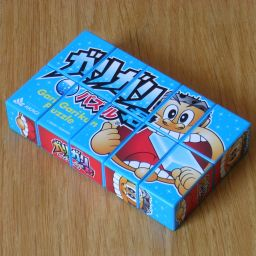# 1×2×5, Gari Garikun PuzzleThe Gari Garikun Puzzle is a 1×2×5 cuboid puzzle. It is like a Rubik's Cube but instead of three layers along each axis, there are 1, 2, and 5 layers, making it a rectangular block instead of a cube. Each layer can rotate, but as the dimensions are all different, only half turns are allowed. The stickers show advertising of an ice lolly of roughly the same size, colour and shape as the puzzle itself.

## The number of positions:

There are two central edge pieces, four wing edge pieces, and four corner pieces. Taking one of the central edge pieces as a reference point, the corners can be permuted in 4! ways, as can the wing edges, while the other central edge can just be flipped or not This gives a total of 2·4!2 = 1,152 positions, all of which can be reached.

I ran a computer search to find out how many moves it takes to solve the puzzle. I have defined a single move as being a twist of the one or two outer layers, so twisting an inner layer on its own is considered two moves.
Depth# Positions
01
15
214
336
495
5200
6298
7292
8166
943
102
Total1,152

This shows that at most 10 moves are needed to solve the puzzle (6.1528 on average).

## Notation:

Hold the puzzle with a large face towards you, and the long axis going left to right. A turn of the top half of the puzzle will be denoted by the letter U. A turn of the outer layer on the left or right will be denoted by L and R respectively, whereas a turn of the outer two layers on the left or right are denoted l and r.

## Solution:

Phase 1: Solve the middle layer.

1. If the top middle edge is flipped relative to the bottom middle edge, then do a U move.

Phase 2: Solve the wing edges.

1. Find the wing edge that belongs on the top right.
2. Depending on where the wing edge lies, do one of the following to solve it:
Top right: Do nothing.
Bottom right: Do r.
Bottom left: Do U l U.
Top left: Do l U l U.
3. Find the wing edge that belongs on the bottom right.
4. Depending on where the wing edge lies, do one of the following to solve it:
Bottom right: Do nothing.
Top left: Do U r U.
Bottom left: Do l U r U.
5. If the two wing edges on the left need to be swapped, do an l move.

Phase 3: Solve the corners.
This is exactly the same as phase 2, except using moves of the outer layers only.

1. Find the corner piece that belongs on the top right.
2. Depending on where the corner lies, do one of the following to solve it:
Top right: Do nothing.
Bottom right: Do R.
Bottom left: Do U L U.
Top left: Do L U L U.
3. Find the corner piece that belongs on the bottom right.
4. Depending on where the corner lies, do one of the following to solve it:
Bottom right: Do nothing.
Top left: Do U R U.
Bottom left: Do L U R U.
5. If the two corners on the left need to be swapped, do an L move.

The pictorial design on the puzzle is very confusing. It is easy to mistake parts of one of the caricature faces for the other. It may therefore sometimes be necessary to fix the centre edges at the end due to a mistake in phase 1 of the solution above.

1. To flip the top middle edge without affecting the other pieces, do r U r U r U.